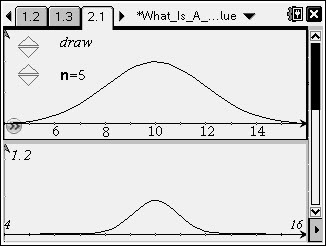# Activities

•##### Download

•• ##### Subject Area

• Math: Statistics: Hypothesis Tests

• ##### Author9-12

45 Minutes

• ##### Device
• TI-Nspire™
• TI-Nspire™ CAS
• ##### Software

TI-Nspire™
TI-Nspire™ CAS

2.1

• ##### Report an Issue

Meaning of P-Value#### Activity Overview

• Students will recognize that a p-value only has meaning if the null hypothesis is true (a conditional probability).
• Students will interpret a p-value in given contexts as the relative frequency for sample statistical values at least as extreme as that from the observed sample, assuming that the null hypothesis is true.
• Students will recognize the relationship between sample size and p-values: in general, for sample means giving p-values less than 0.5, increasing the sample size decreases the p-value.

#### Key Steps

•Students begin by looking at a population with a mean of 10, which is assumed in the null hypothesis. They will use an animation to draw various sizes of samples from the population.